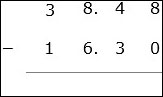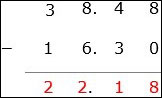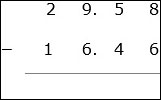# Decimal Subtraction: Basic

Basic subtraction of decimals is similar to subtraction of whole numbers without borrowing.

• First the decimals are written in such a way that digits with same place values are vertically aligned.

• We then add zeros to the right of decimal numbers where ever necessary so that all numbers have the same number of digits after the decimal point.

• We begin with the rightmost column and start subtracting the digits. Then we keep moving to the left till all columns are subtracted.

Subtract 38.48 − 16.3

### Solution

Step 1The decimals are aligned as shown. A zero is added to the right of 16.3 as the maximum number of decimal places is 2.

Step 2

We start with the right most column. Subtract 8 − 0 = 8; then moving to left column 4 − 3 = 1; again moving left 8 − 6 = 2; next 3 − 1 = 2;

Step 3

So the difference of the decimals is 12.18Subtract 29.58 − 16.46

### Solution

Step 1The decimals are aligned as shown.

Step 2

We start with the right most column. Subtract 8 − 6 = 2; then moving to left column 5 − 4 = 1; again moving left 9 − 6 = 3; next 2 − 1 = 1;

Step 3

So the difference of the decimals is 13.12